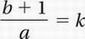# SAT Math Multiple Choice Question 655: Answer and Explanation

### Test Information

Question: 655

10. The equation y = kx - 1, where k is a constant, describes a line in the xy-plane. If the graph of this line contains the point (a, b), where a and b are nonzero, what is the value of k in terms of a and b?

• A.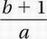• B.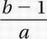• C.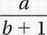• D.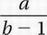Explanation:

A

Algebra (linear equations) MEDIUM

Original equation:

y = kx - 1

Substitute x 5 a and y 5 b:

y = ka - 1